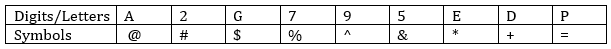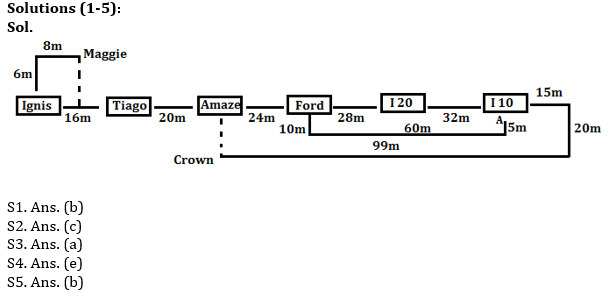Latest Banking jobs   »   Reasoning Ability Quiz For RRB PO...   »   Reasoning Ability Quiz For RRB PO...

# Reasoning Ability Quiz For RRB PO Mains 2021- 16th September

Directions (1-5): Study the following information carefully and answer the questions given below.
Six cars I 10, Ignis, Tiago, I 20, Amaze, Ford are parked in a row facing north at a distance which is a successive multiple of 4m in increasing order from the left end from each other. Ford is second to the right of Tiago. The total distance between Amaze and I 20 is 52m. Only one car is parked in between Ignis and Amaze. Ford and I 20 are parked next to each other. I 10 and Ford are not parked next to Ignis. Now Car Ignis starts moving towards north direction after moving 6m it takes a right turn and stops at Maggie point after moving 8m. Car I 10 starts moving in east direction and after going 15m it turns right and move 20m and then again turn right and move 99m and stops there at Crown point. Ford car starts moving in south direction and after moving 10m it takes a left turn and moves 60m then it again takes a left turn and moves 5m and stop at Point A.

Q1. What is the distance and direction of initial position of Car ‘Ignis’ with respect to the initial position of Car ‘Amaze’?
(a) 36m, East
(b) 36m, West
(c) 34m, North-west
(d) 60, East
(e) None of these

Q2. What is the shortest distance between Maggie point and Initial position of car ‘Ignis’?
(a) 16m
(b) 20m
(c) 10m
(d) 36m
(e) 44m

Q3. What is the total distance between initial position of cars ‘Tiago’ and ‘I10’?
(a) 104m
(b) 121m
(c) 84m
(d) 44m
(e) 108m

Q4. How many cars are parked there between cars ‘Amaze’ and “Ford’?
(a) Two
(b) Three
(c) More than three
(d) One
(e) None

Q5. What is the difference between the total distance of initial and final position of car ‘I10’ and the total distance of initial and final position of car ‘Ford’?
(a) 56m
(b) 59m
(c) 53m
(d) 54m
(e) 50m

Directions (6-9): In each of the questions given below, a group of digits is given followed by four combinations of symbols numbered (a), (b), (c) and (d). You have to find out which of the four combinations correctly represents the group of digits based on the symbol codes and the conditions given below. If none of the four combinations represents the group of digits correctly, give (e) ‘None of these’ as the answer.Conditions for coding-
(1) If the first element is even number and the last element is vowel then both are to be coded as the code of vowel.
(2) If the first element is odd number and last element is consonant then both are to be coded as the code of the last element.
(3) If the first element is vowel and the last element is consonant then the codes of both elements are to be interchanged.
(4) If the first element is consonant and last element is even number then both are to be coded as the code of the last element.

Q6. DEG7A2
(a) #*\$%@#
(b) +*\$%@+
(c) #*\$@%#
(d) #*\$%@+
(e) None

Q7. 9DE52P
(a) =*+&#=
(b) ^+*&#^
(c) =+*&#=
(d) =+*#&^
(e) None

Q8. 2G795A
(a) @\$^%&@
(b) @\$%^&@
(c) #\$%^&#
(d) @4%^&#
(e) None

Q9. E2PD9G
(a) *#=+^\$
(b)\$ #=+^\$
(c)* #=+^*
(d) \$#=+^*
(e) None

Directions (10): In the given question below is given a statement followed by two conclusions numbered I and II. You have to assume everything in the statement to be true, then consider the two conclusions together and decide which of them logically follows beyond a reasonable doubt from the information given in the statement.

Q10. Statement: Irregularity is a cause for failure in exams. Some regular students fail in the examinations.
Conclusions:
I. All failed students are regular.
II. All successful students are not regular.
(a) if only conclusion I follows;
(b) if only conclusion II follows;
(c) If either I or II follows;
(d) if neither I nor II follows; and
(e) if both I and II follow.

SolutionsS6. Ans.(a)
Sol. Here, condition (4) is used

S7. Ans.(c)
Sol. Here, condition (2) is used

S8. Ans.(b)
Sol. Here, condition (1) is used

S9. Ans.(d)
Sol. Here, condition (3) is used

S10.Ans. (d)
Sol. The-given statement clearly implies that all irregular and some regular students fail in the examinations. This, in turn, means that all successful students are regular but not all regular students are successful. So, neither I nor II follows.

Click Here to Register for Bank Exams 2021 Preparation Material#### Congratulations!Download Hindu Review of October 2021: Free PDF